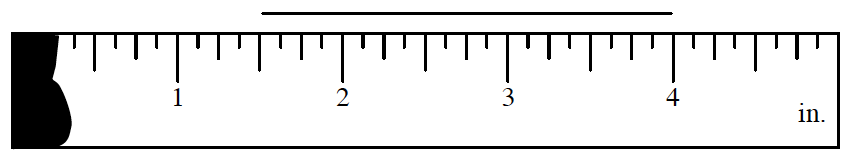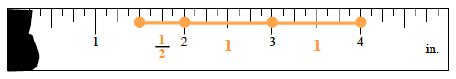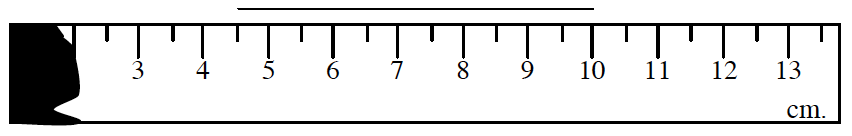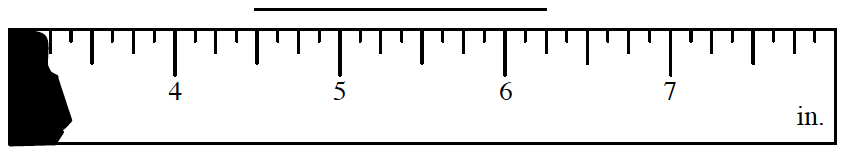### Home > MC1 > Chapter 2 > Lesson 2.1.1 > Problem2-8

2-8.

Use the painted rulers below to measure the length of each line segment.

1.Try to remember how you found the length of the rulers Ms. Mikles took in problem 2-2 in class.

One way is to count the whole parts and then the leftover parts. For this problem, count two whole inches (one from $2$ to $3$ and the other from the $3$ to $4$). Then, count the half inch (from $1.5$ to $2$).2.This one is just like the one above. Remember to count the whole centimeters and then the partial centimeters.

3.Try to count the number of whole inches and then the number of quarter inches and half inches.